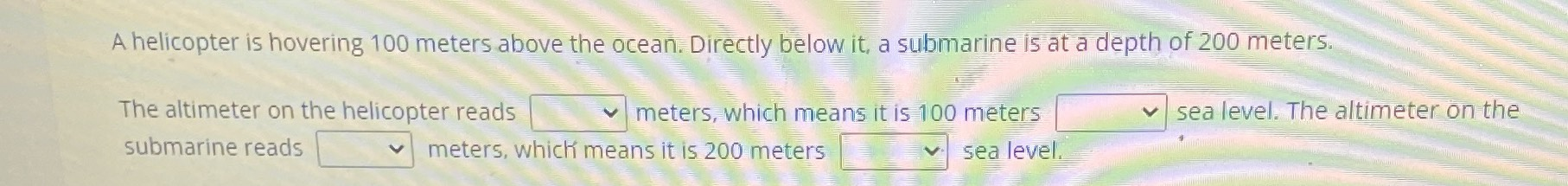### ¿Todavía tienes preguntas de matemáticas?

Pregunte a nuestros tutores expertos
Algebra
PreguntaA helicopter is hovering $$100$$ meters above the ocean. Directly below it, a submarine is at a depth of $$200$$ meters. The altimeter on the helicopter reads $$\square _ { v }$$ meters, which means it is $$100$$ meters $$\square$$ sea level. The altimeter on the submarine reads $$\square$$ seans it is $$200$$ meters $$\square$$ sever.